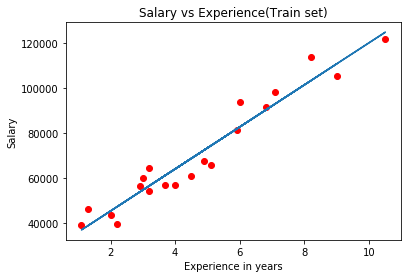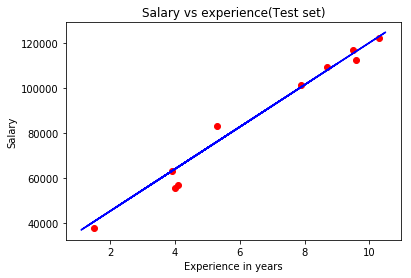×

Search anything:

# Linear regression in Python using Scikit Learn

#### Machine Learning (ML) PythonFREE BOOK -> Problems for the day before your Coding Interview (on Amazon)

Reading time: 25 minutes | Coding time: 10 minutes

In this article, we will implement linear regression in Python using scikit-learn and create a real demo and get insights from the results.

First of all, we shall discuss what is regression.

Regression

The statistical methods which helps us to estimate or predict the unknown value of one variable from the known value of related variable is called regression.

Determing the line of regression means determining the line of best fit.

Linear Regression

Linear regression is an algorithm that assumes that the relationship between two elements can be represented by a linear equation (y=mx+c) and based on that, predict values for any given input. It looks simple but it powerful due to its wide range of applications and simplicity.

Scikit-learn

Scikit-learn is a popular machine learning library for Python and supports several operations natively like classification, regression, clustering and includes a wide variety such as DBSCAN and gradient boosting. It is designed to better use NumPy and SciPy libraries of Python.

The mathematicl equation for linear regression is

``````y= a + bx
``````

here y is the dependent variable which we are going to predict.
a is the constant term, and b is the coeffient and x is the independent variable.
For the example given below the equation can be stated as

``````Salary = a + b * Experience
``````

Now we will see simple linear regression in python using scikit-learn

Here is the code:

``````import numpy as np
import pandas as pd
import matplotlib.pyplot as plt
%matplotlib inline
``````

Importing the libraries numpy for linear algebra matrices, pandas for dataframe manipulation and matplotlib for plotting and we have written %matplotlib inline to view the plots in the jupyter notebook itself.

``````#importing the data set
``````

here we are importing a dataset which contains two columns years of experience and salary.

You can check the data by typing this

``````dataset.info
``````

now we divide our dataset into X and y, where X is the independent variable and y is the dependent variable.

``````X=dataset.iloc[:,:-1].values
y=dataset.iloc[:,1].values
``````

Now we will split our data into training set and test set.
Training set is the one on which we train our model and test set is one by which we test our model by giving it new values to see if our model predicts correctly or not.

``````from sklearn.model_selection import train_test_split
X_train,X_test,y_train,y_test=train_test_split(X,y,test_size=1/3,random_state=0)
``````

Here test_size means how much of the total dataset we want to keep as our test data.
and random_state=0 so that your output is same as mine.

Now we will fit linear regression model t our train dataset

``````from sklearn.linear_model import LinearRegression
regressor=LinearRegression()
regressor.fit(X_train,y_train)
``````

Here LinearRegression is a class and regressor is the object of the class LinearRegression.And fit is method to fit our linear regression model to our training datset.

Now its time to predict our results

``````y_pred=regressor.predict(X_test)
``````

Now you can check both y_test and y_pred to see if our linear model predicts salary value near to the original salary value.

Now we will visualize our training set results

``````#Visualsing the training set results
plt.scatter(X_train,y_train,color='red')
plt.plot(X_train,regressor.predict(X_train),)
plt.title('Salary vs Experience(Train set)')
plt.xlabel('Experience in years')
plt.ylabel('Salary')
``````Now we will visualize the test set results

``````#Visualising the test set results
plt.scatter(X_test,y_test,color='red')
plt.plot(X_train,regressor.predict(X_train),color='blue')
plt.title('Salary vs experience(Test set)')
plt.xlabel('Experience in years')
plt.ylabel('Salary')
plt.show()
``````Our linear regression models's prediction are quite good, all the predictions are quite near to the original values.

## When to use Linear regression model ?

We use linear model when we see a linear relationship between the dependent and independent variables.

Example test cases for linear regression model:

1. Sales of a product; pricing, performance, and risk parameters.
2. Generating insights on consumer behavior, profitability, and other business factors.
3. Evaluation of trends; making estimates, and forecasts.
4. Determining marketing effectiveness, pricing, and promotions on sales of a product.
5. Assessment of risk in financial services and insurance domain.
6. Studying engine performance from test data in automobiles.

## Linear Regression VS Logistic Regression

Linear regression
It is a regression algorithm in which we predict a continous value.

Logistic regression
It is a classification algorithm, it returns either positive or negative value (in case of binary classification).